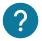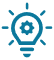# What is a string of characters? How to do operations with it? How to convert into strings?

## Introduction

A string of characters is a variable used to store any types of  character from the ASCII table.Thanks to this type of variable, you can write messages.

Here is how to use the string of characters:

``char String[] = "arduino factory";``### What is an ASCII table?

The ASCII table contains all the characters that you can use in your string character variable.

This is the table of all the ASCII characters:This table can be scary, but we will only use the char’s column. In fact, in this column you will find all the characters you can use in your string char variable.### Can we add or subtract the strings of char?

The string of characters are a particular variable because we can’t add or subtract them with + or -. Nevertheless you can concatenate two strings of characters to gather them.

If you want to add two string of characters together, you can use the string variables.### The numbers in a string of characters

If you put numbers in a string of characters, these ones will be treated as characters and not as a mathematical number. If you have only numbers in your string of characters, you can convert it to an integer variable:

``````char char_variable[] = "45"; // Here is the number in string
int integer_variable = atoi(char_variable); // Here is a mathematical number``````

By converting your number into an integer, you will have the possibility to add, subtract or do others mathematical operations.### How to initialize multiple messages in only one string of characters?

If you want to write many messages in a the same variable, you will need a list of characters.

Here is an example:

``````char *myStrings[] = {"This is string 1", "This is string 2", "This is string 3",
"This is string 4", "This is string 5", "This is string 6"
};
``````

To know how to display one message, or to do some operations on this list, you can read our course about the lists.